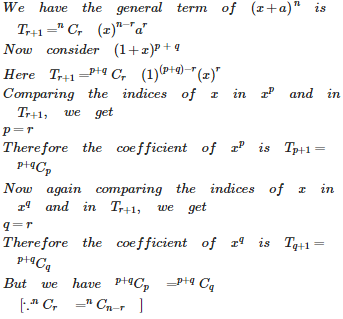# Binomial Theorem - Online Test

Q1.

If n is a rational number, which is not a whole number, then the number of terms in the expansion of  is

Explaination / Solution:Q2.

If n is a +ve integer, then the binomial coefficients equidistant from the beginning and the end in the expansion of  are

Explaination / Solution: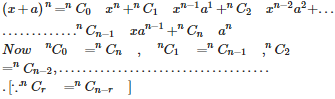Q3.

If the rth term in the expansion of  contains  then r =

Explaination / Solution:Q4.

If the coefficients of  and  in the expansion of  are equal then n =

Explaination / Solution: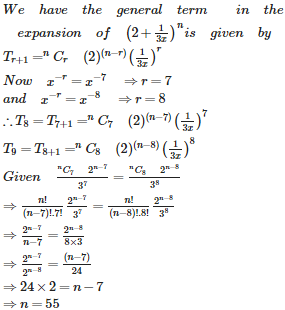Q5. The largest term in the expansion of  when  is
Explaination / Solution: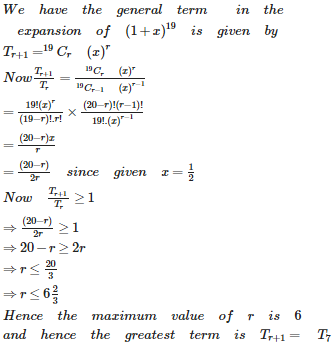Q6. The exponent of  x occurring in the  term of expansion of  is
Explaination / Solution:Q7. The term independent of x in the expansion of  is
Explaination / Solution: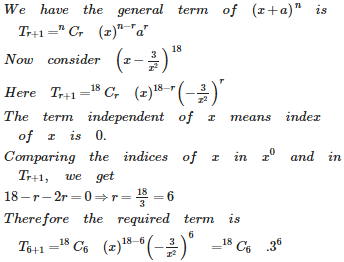Q8. The number of dissimilar terms in the expansion of is
Explaination / Solution: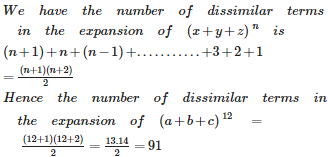Q9. The term containing  in the expansion of  is
Explaination / Solution: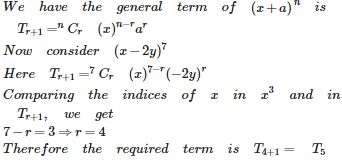Q10. The coefficients of  and  ( p, q are + ve integers) in the binomial expansion of  are
Explaination / Solution: